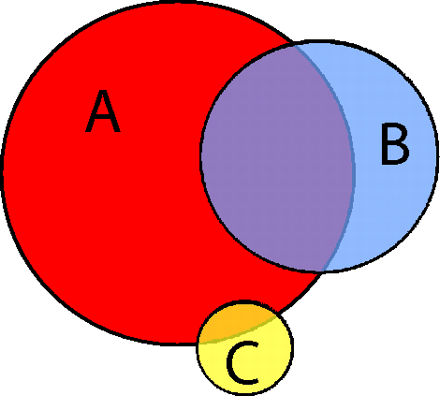### Generalized Venn diagram for genetics

by @ulaulaman http://t.co/MkGI7L546N #VennDay #VennDiagram #geneticsA generalized Venn diagram with three sets $A$, $B$ and $C$ and their intersections. From this representation, the different set sizes are easily observed. Furthermore, if individual elements (genes) are contained in more than one set (functional category), the intersection sizes give a direct view on how many genes are involved in possibly related functions. During optimization, the localization of the circles is altered to satisfy the possibly contradictory constraints of circle size and intersection size.
For the purpouse of the paper, the researchers used polygons instead of circles. In order to compute the polygons' area, they used the simple formula: $A = \sum_{k=1}^L x_k (y_{k+1} - y_k)$ where $L$ is the number of the edges of the polygon, and $y_{L+1} := y_1$.
Kestler, H., Muller, A., Gress, T., & Buchholz, M. (2004). Generalized Venn diagrams: a new method of visualizing complex genetic set relations Bioinformatics, 21 (8), 1592-1595 DOI: 10.1093/bioinformatics/bti169